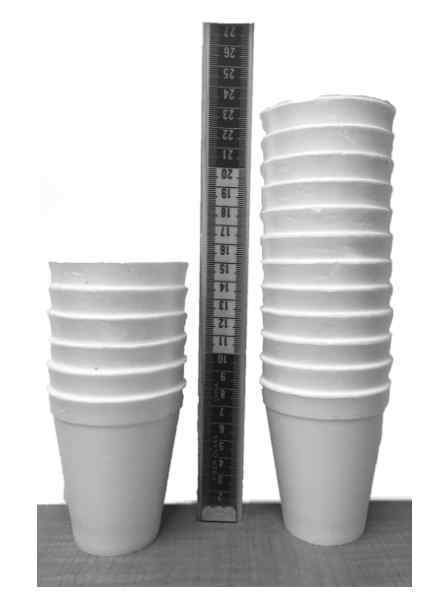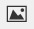PSABM6V2 - Student Facing Task---Acc7.5 Lesson 4 Introduction to Linear Relationships (6.NS.A, 7.RP.A.2.a, 8.EE.B)

Find the value ofPart A)

We have two stacks of styrofoam cups. One stack has 6 cups, and its height is 15 cm. The other one has 12 cups, and its height is 23 cm.

How many cups are needed for a stack with a height of 50 cm?Type your answer below as a number (example: 5, 3.1, 4 1/2, or 3/2):
Part B)

If you didn’t create your own graph of the situation before, do so now.

Draw your graph on paper, take a picture, and upload it using the image upload iconIf you do not have the ability to upload an image of your work, type "Graph is on paper."

Part C)

What are some ways you can tell that the number of cups is not proportional to the height of the stack?

Part D)

What is the slope of the line in your graph?

Part E)

What does the slope mean in this situation?

Part F)

At what point does your line intersect the vertical axis?

(x,y)

with no spaces.

Part G)

What do the coordinates of this point tell you about the cups?